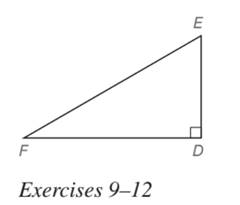Chapter 5.5, Problem 9EElementary Geometry For College St...

7th Edition
Alexander + 2 others
ISBN: 9781337614085

Solutions

Chapter
SectionElementary Geometry For College St...

7th Edition
Alexander + 2 others
ISBN: 9781337614085
Textbook Problem

In Exercises 5 to 22, find the missing lengths. Give your answers in both simplest radical form and as approximations correct two decimal places. Given: Right ∆ D E F with m ∠ E = 60 ° and D E = 5 Find: D F and F ETo determine

To find:

DF and FE of the right DEF with mE=60° and DE=5.

Explanation

Approach:

For a right triangle, for which the measure of the interior angles 30°, 60°, and 90°; if ‘a’ is the length of measure of the shorter leg; opposite to the angle 30°, then the length of the other two sides is given by

Length of the longer leg (opposite to 60°) =a3

Length of the hypotenuse (opposite to 90°) =2a.

In general

Length of the longer leg =3× (Length of the shorter leg)

Length of the hypotenuse =2× (Length of the shorter leg)

Calculation:

Given,

A right triangle DEF with mE=60° and DE=5.

Since, one of the acute angle mE of the right triangle is 60°, then the other acute angle mF should be 30°.

Thus, the XYZ is of the type 30°-60°-90° triangle.

30°-60°-90° theorem.

In a right triangle whose angle measure 30°, 60°, and 90°, the hypotenuse has a length equal to twice the length of the shorter leg, and the longer leg is the product of 3 and the length of the shorter leg

Still sussing out bartleby?

Check out a sample textbook solution.

See a sample solution

The Solution to Your Study Problems

Bartleby provides explanations to thousands of textbook problems written by our experts, many with advanced degrees!

Get Started

1. If , find y’.

Mathematical Applications for the Management, Life, and Social Sciences

In Exercises 99-106, factor out the greatest common factor from each expression. 103. ex xex

Applied Calculus for the Managerial, Life, and Social Sciences: A Brief Approach

Which trigonometric functions are even?

Trigonometry (MindTap Course List)

True or False: converges conditionally.

Study Guide for Stewart's Multivariable Calculus, 8th

Evaluate ∫16t3 ln t dt. 4t4 + C 4t3 ln t − 16t3 + C 4t4 − t3 ln t + C 4t4 ln t − t4 + C

Study Guide for Stewart's Single Variable Calculus: Early Transcendentals, 8th